Previous Page | Next Page

 The MCMC Procedure
 The Behrens-Fisher Problem

One of the famous examples in the history of statistics is the Behrens-Fisher problem (Fisher; 1935). Consider the situation where there are two independent samples from two different normal distributions: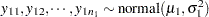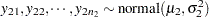Note that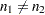. When you do not want to assume that the variances are equal, testing the hypothesis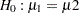is a difficult problem in the classical statistics framework, because the distribution under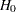is not known. Within the Bayesian framework, this problem is straightforward because you can estimate the posterior distribution of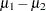while taking into account the uncertainties in all of parameters by treating them as random variables.

Suppose that you have the following set of data:

```title 'The Behrens-Fisher Problem';

data behrens;
input y ind @@;
datalines;
121 1  94 1 119 1 122 1 142 1 168 1 116 1
172 1 155 1 107 1 180 1 119 1 157 1 101 1
145 1 148 1 120 1 147 1 125 1 126 2 125 2
130 2 130 2 122 2 118 2 118 2 111 2 123 2
126 2 127 2 111 2 112 2 121 2
;
```

The response variable is y, and the ind variable is the group indicator, which takes two values: 1 and 2. There are 19 observations that belong to group 1 and 14 that belong to group 2.

The likelihood functions for the two samples are as follows: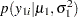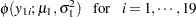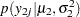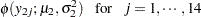Berger (1985) showed that a uniform prior on the support of the location parameter is a noninformative prior. The distribution is invariant under location transformations—that is,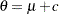. You can use this prior for the mean parameters in the model: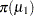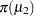In addition, Berger (1985) showed that a prior of the form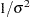is noninformative for the scale parameter, and it is invariant under scale transformations (that is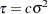). You can use this prior for the variance parameters in the model: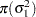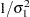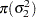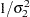The log densities of the prior distributions on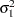and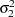are: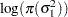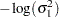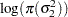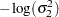The following statements generate posterior samples of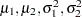, and the difference in the means::

```proc mcmc data=behrens outpost=postout seed=123
nmc=40000 thin=10 monitor=(_parms_ mudif)
statistics(alpha=0.01)=(summary interval);
ods select PostSummaries PostIntervals;
parm mu1 0 mu2 0;
parm sig21 1;
parm sig22 1;
prior mu: ~ general(0);
prior sig21 ~ general(-log(sig21));
prior sig22 ~ general(-log(sig22));
mudif = mu1 - mu2;
if ind = 1 then
llike = lpdfnorm(y, mu1, sqrt(sig21));
else
llike = lpdfnorm(y, mu2, sqrt(sig22));
model general(llike);
run;
```

The PROC MCMC statement specifies an input data set (behrens), an output data set containing the posterior samples (postout), a random number seed, the simulation size, and the thinning rate. The MONITOR= option specifies a list of symbols, which can be either parameters or functions of the parameters in the model, for which inference is to be done. The symbol _parms_ is a shorthand for all model parameters—in this case, mu1, mu2, sig21, and sig22. The symbol mudif is defined in the program as the difference between
and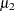.

The ODS SELECT statement displays the summary statistics and interval statistics tables while excluding all other output. For a complete list of ODS tables that PROC MCMC can produce, see the sections Displayed Output and ODS Table Names.

The STATISTICS= option calculates summary and interval statistics. The global suboption ALPHA=0.01 specifies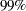equal-tail and highest posterior density (HPD) credible intervals for all parameters.

The PARMS statements assign the parameters mu1 and mu2 to the same block, and sig21 and sig22 each to their own separate blocks. There are a total of three blocks. The PARMS statements also assign an initial value to each parameter.

The PRIOR statements specify prior distributions for the parameters. Because the priors are all nonstandard (uniform on the real axis forandandforand), you must use the GENERAL function here. The argument in the GENERAL function is an expression for the log of the distribution, up to an additive constant. This distribution can have any functional form, as long as it is programmable using SAS functions and expressions. Note that the function specifies a distribution on the log scale, not the original scale. The log of the prior on mu1 and mu2 is, and the log of the priors on sig21 and sig22 are -log(sig21) and -log(sig22) respectively. See the section Specifying a New Distribution for more information about how to specify an arbitrary distribution.

The mudif assignment statement calculates the difference between mu1 and mu2. The IF-ELSE statements enable different y’s to have different log-likelihood functions, depending on their group indicator ind. The function LPDFNORM is a PROC MCMC function that calculates the log density of a normal distribution. See the section Using Density Functions in the Programming Statements for more details. The MODEL statement specifies that llike is the log likelihood for each observation in the model.

Figure 52.7 displays the posterior summary and interval statistics.

Figure 52.7 Posterior Summary and Interval Statistics
 The Behrens-Fisher Problem

The MCMC Procedure

Posterior Summaries
Parameter N Mean Standard
Deviation
Percentiles
25% 50% 75%
mu1 4000 134.8 6.0065 130.9 134.7 138.7
mu2 4000 121.4 1.9150 120.2 121.4 122.7
sig21 4000 683.2 259.9 507.8 630.1 792.3
sig22 4000 51.3975 24.2881 35.0212 45.7449 61.2582
mudif 4000 13.3596 6.3335 9.1732 13.4078 17.6332

Posterior Intervals
Parameter Alpha Equal-Tail Interval HPD Interval
mu1 0.010 118.7 150.6 119.3 151.0
mu2 0.010 115.9 126.6 116.2 126.7
sig21 0.010 292.0 1821.1 272.8 1643.7
sig22 0.010 18.5883 158.8 16.3730 140.5
mudif 0.010 -3.2537 29.9987 -3.1915 30.0558

The mean difference has a posterior mean value of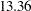, and the lower endpoints of thecredible intervals are negative. This suggests that the mean difference is positive with a high probability. However, if you want to estimate the probability that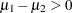, you can do so as follows.

The following statements produce Figure 52.8:

```proc format;
value diffmt low-0 = 'mu1 - mu2 <= 0' 0<-high = 'mu1 - mu2 > 0';
run;

proc freq data = postout;
tables mudif /nocum;
format mudif diffmt.;
run;
```

The sample estimate of the posterior probability thatis 0.98. This example illustrates an advantage of Bayesian analysis. You are not limited to making inferences based on model parameters only. You can accurately quantify uncertainties with respect to any function of the parameters, and this allows for flexibility and easy interpretations in answering many scientific questions.

Figure 52.8 Estimated Probability of.
 The Behrens-Fisher Problem

The FREQ Procedure

mudif Frequency Percent
mu1 - mu2 <= 0 77 1.93
mu1 - mu2 > 0 3923 98.08Previous Page | Next Page | Top of Page